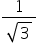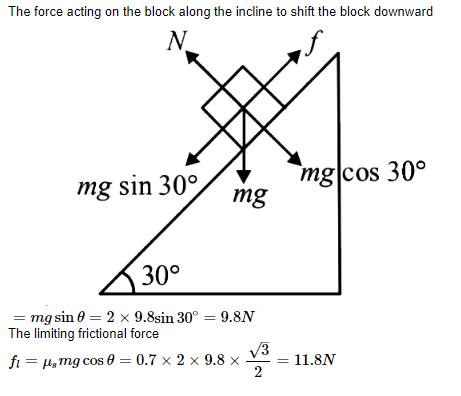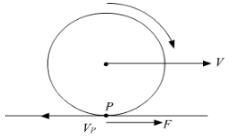Courses

# Test: Friction

## 10 Questions MCQ Test Physics Class 11 | Test: Friction

Description
This mock test of Test: Friction for JEE helps you for every JEE entrance exam. This contains 10 Multiple Choice Questions for JEE Test: Friction (mcq) to study with solutions a complete question bank. The solved questions answers in this Test: Friction quiz give you a good mix of easy questions and tough questions. JEE students definitely take this Test: Friction exercise for a better result in the exam. You can find other Test: Friction extra questions, long questions & short questions for JEE on EduRev as well by searching above.
QUESTION: 1

### Complete the sentence. Friction always ____________

Solution:

Frictional forces act over the common surfaces of the two bodies to avoid and restrict relative moment between those two surfaces.

QUESTION: 2

### After the body starts moving, the friction involved with motion is

Solution:

When the body is in rest it is under static friction but when it starts moving (neither rolling nor sliding), the static friction slowly chngs to kinetic friction as the coefficient of static friction start decreasing and that of kinetic friction starts increasing. In case it starts rolling motion then the friction is rolling friction & if it slides then sliding fiction.

QUESTION: 3

### Impending motion of a body is opposed by

Solution:

The impending motion refers to the state of a body when it is on the verge of slipping. In such cases, the static friction has reached its upper limit and is given by the equation, F=μsN

QUESTION: 4

A block of mass 1 kg lies on a horizontal surface in a truck. The coefficient of static friction between the block and the surface is 0.6. If the acceleration of the truck is 5 ms-2, the frictional force acting on the block is

Solution:

Limiting friction, f = μmg = 0.6 × 1 × 9.8 = 5.88 N

Applied force = F = ma = 1 × 5 = 5 N

As F < f, so force of friction = 5 N

QUESTION: 5

A particle moves in a circle with a constant speed of 6m /s. Its centripetal acceleration is 120 m/s2, The radius of the circle is

Solution:

We know that centripetal acceleration, c = v2/r
Thus we get r = v2/c
= 36 / 120
= .3 /m

QUESTION: 6

A body rests on an inclined plane and the angle of inclination is varied till the body just begins to slide down. The coefficient of friction is. What is the angle of inclination?

Solution:

At the time when the block just starts to move, we get that net force acting upon it is 0, thus we get, f - mg.sin a = 0
Where f is friction force and a is angle of incline.
We also know that f = 1√3 x N
= 1√3 x mg.cos a
Thus we get 1√3 x mg.cos a = mg.sin a
Thus we get tan a = 1√3
And a = 30

QUESTION: 7

A block of mass 2kg rests on a plane inclined at an angle of 30with the horizontal. The coefficient of friction between the block and the surface is 0.7. The frictional force acting on the block is

Solution:QUESTION: 8

A particle moves in a circle of radius 0.30m with a constant speed of 6m/s. Its centripetal acceleration is

Solution:

We know that centripetal acceleration, a = v x v /r
Here v = 6m/s and r = 0.3m
Thus a = 36 / 0.3 m/s2
= 120 m/s2

QUESTION: 9

When a wheel rolls on a level road, the direction of frictional force at the point of contact of wheel and ground is

Solution:What you need to remember and apply here is that friction always tries to oppose motion.
So, in case of the wheel, the point P which is in contact with the ground tries to go backwards due to rotation and it's velocity as shown in the figure.
The frictional force F will try to oppose this motion and thus, it will be in the forward direction.

QUESTION: 10

A force of 20 N is applied on a body of mass 4 kg kept on a rough surface having coefficient of friction 0.1. Find acceleration of body. Take g = 10 m/s2

Solution:

The normal force due to the weight of the bloch is 40N, hence the friction force is 4N acting to restrict the motion.
Hence the net force on the body is thus 20 - 4 = 16N
Thus the net acceleration of the block is 16/4 = 4ms2